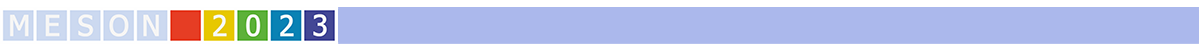#MESON2023

Jun 22 – 27, 2023
Auditorium Maximum
Europe/Warsaw timezone

## Accessing the strong interaction between $\Lambda$ baryons and kaons with femtoscopy at LHC

Jun 24, 2023, 3:00 PM
20m
Conference room (Auditorium Maximum)

Parallel

### Speaker

Valentina Mantovani Sarti (Technical University of Munich)

### Description

The interaction between $\Lambda$ baryons and kaons/antikaons is a crucial ingredient for low energies effective models aiming at describing the strangeness $S=0$ ($\Lambda$K) and $S=-2$ ($\Lambda$$\mathrm{\bar{K}}) sector of the meson-baryon interaction. Of particular interest is the \Lambda$$\mathrm{\bar{K}}$ interaction, where the presence of the \XRes close to the threshold should play a significant role. The $\pi\Xi$ decay channel of the $\Xi(1620)$ resonance has been recently observed by the Belle collaboration, providing the first experimental values for its mass and width. However, no measurements are available for the $\Lambda$$\mathrm{\bar{K}} channel. Delivering high-precision data on the \Lambda$$\mathrm{\bar{K}}$ system can help to shed light into the nature of the $\Xi(1620)$ state and its formation. Experimental data on $\Lambda$$\mathrm{\bar{K}}, and as well on \LambdaK, are currently rather scarce, leading to large uncertainties and tensions between the available theoretical predictions. Recently, measurements of two-particle correlations in small colliding systems such as pp collisions at the LHC provided a significant improvement in the knowledge of several hadron-hadron interactions, in particular when strange baryons and mesons are involved. In this talk, we present \LambdaK^+ and \LambdaK^- femtoscopic correlations measured by ALICE in pp collisions at \sqrt{s}=13 TeV, obtained with a high-multiplicity trigger. The measurements indicate a repulsive interaction for \LambdaK^+ pairs and an attractive interaction for \LambdaK^-. In particular, we will show the first experimental evidence of the \Xi(1620) decaying into \LambdaK^- pairs and discuss the effect of the \Xi(1620) state on the \Lambda$$\mathrm{\bar{K}}$ interaction.

Collaboration ALICE

### Primary author

Valentina Mantovani Sarti (Technical University of Munich)